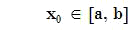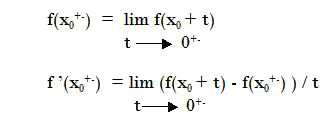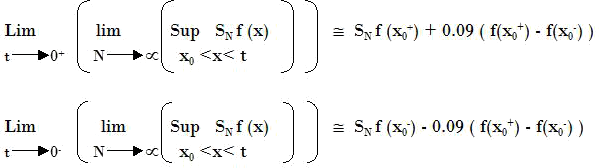# Convergence of Fourier Series discontinuities

### Basic Concepts and PrinciplesFourier series convergence to the midjump-value in jump-discontinuities points.
We will see at this section what is the behavior of the Fourier series for those functions with jump-discontinuities.
Exactly, the Dirichlet theorem assures us that the jump discontinuities, the Fourier series converges to the middle value of jump.
We also study the so-called Gibbs Phenomenon, which says that we are near a jump discontinuity, the Fourier series firing a 9% jump in the value of the jump.

### Extended Theory

Theorem (Dirichlet)
Let f be a continuous function except at a pointSuppose there exitsThen the Fourier series converges to the midpoint of the jump, ieWe will now see the so-called Gibbs Phenomenon
Theorem (Gibbs Phenomenon)
Let f be as in the previous theorem, then the Fourier series of f near ofIt shoots with a value of approximately 9% of the value of the jump of f, ie## Was useful? want add anything?

Post here

### Post from other users

Post here
Update cookies preferences